Get instant live expert help with Excel or Google Sheets“My Excelchat expert helped me in less than 20 minutes, saving me what would have been 5 hours of work!”

#### Post your problem and you’ll get Expert help in seconds.

Your message must be at least 40 characters
Our professional Expert are available now. Your privacy is guaranteed.

# How to Combine SUMIF with GREATER THAN and LESS THAN Functions

When we have to add data based on a given criteria in Excel, the SUMIF function provides the perfect solution. In this article, we will learn how to use SUMIF in combination with Greater Than and Less Than functions.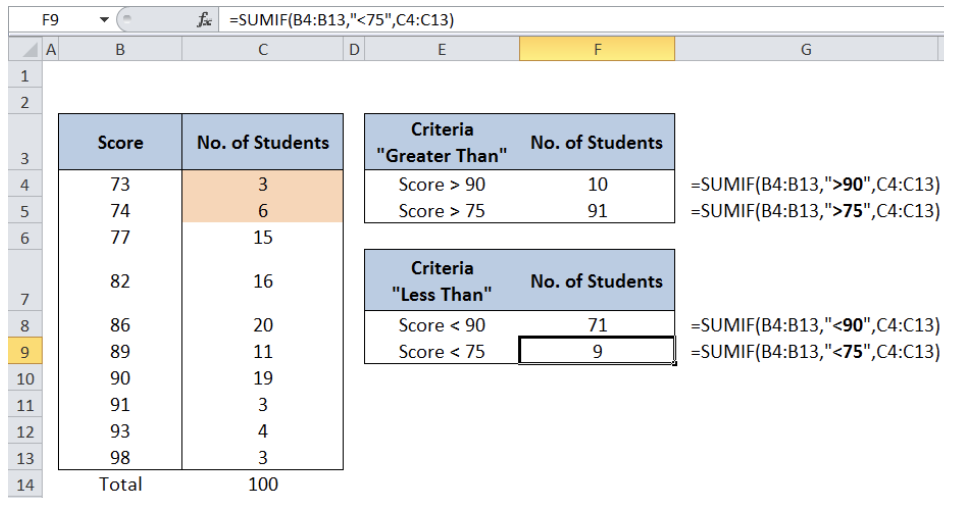Figure 1.   SUMIF with greater than and less than functions

## Setting up the Data

Here we have a table of Scores from 73 to 98 and the corresponding number of students for each score.  We want to know the total number of students who had the following scores:

• Greater than 90
• Greater than 75
• Less than 90
• Less than 75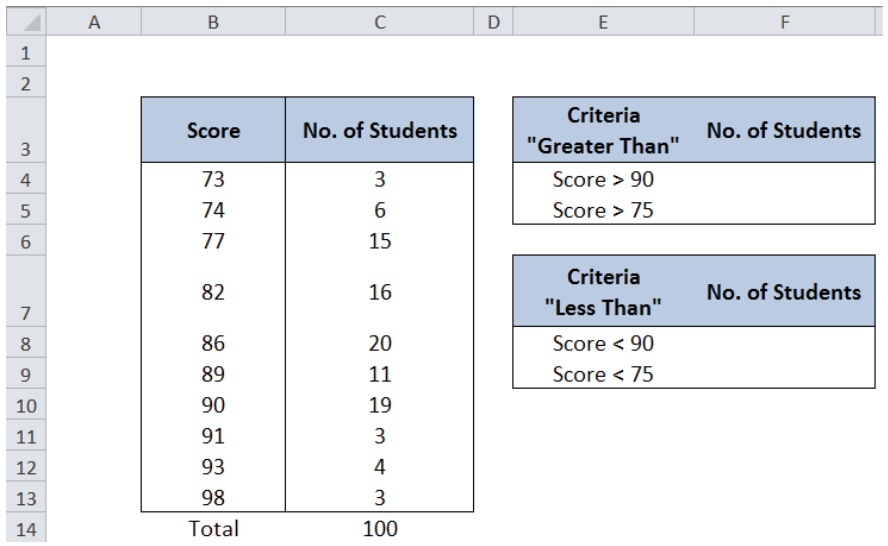Figure 2.  Sample table for SUMIF with Greater than and Less Than Functions

## SUMIF function in Excel

SUMIF is a function that sums the values in a specified range, based on a given criteria.

### Syntax

`=SUMIF(range, criteria, [sum_range])`

Where

• Range: the data range that will be evaluated using the criteria
• Criteria: the criteria or condition that determines which cells will be added
• Sum_range: the cells that will be added; if left blank, “sum_range” = “range” which means that the range of data that will be added is the same range of data evaluated

## Combine SUMIF with Greater Than Function

### Example 1:

We want to know how many students had a score greater than 90.

In cell F4, enter the formula =SUMIF(B4:B13,”>90″,C4:C13).

Where

• B4:B13 is the range that we want to evaluate, containing the scores

• “>90” is the working formula to determine the scores greater than 90
• C4:C13 is the range that contains the data that we want to add, which is the number of students who have scores greater than 90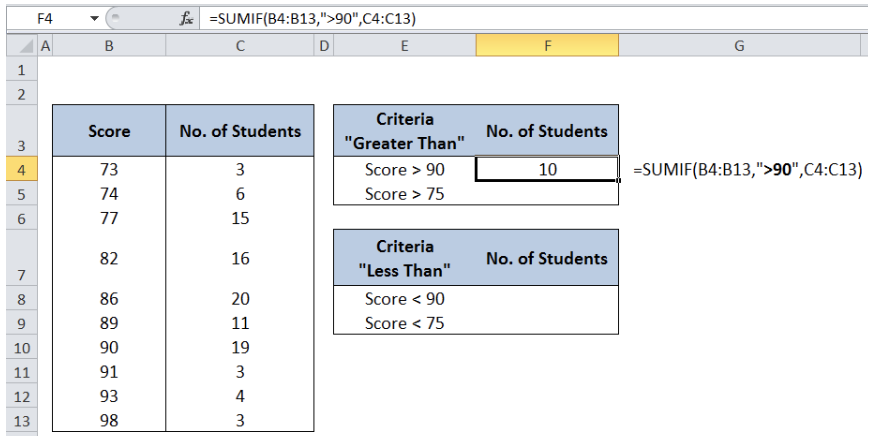Figure 3.  Entering the formula with SUMIF and Greater Than Function

Interpretation of formula: Compute the sum of Column C (No. of Students) if Column B (Score) is greater than 90

The result is 10 students. As shown below, the number of students with scores greater than 90 are highlighted in orange.  The sum of 3, 4 and 3 is 10, which corresponds to the number of students who scored 91, 93 and 98.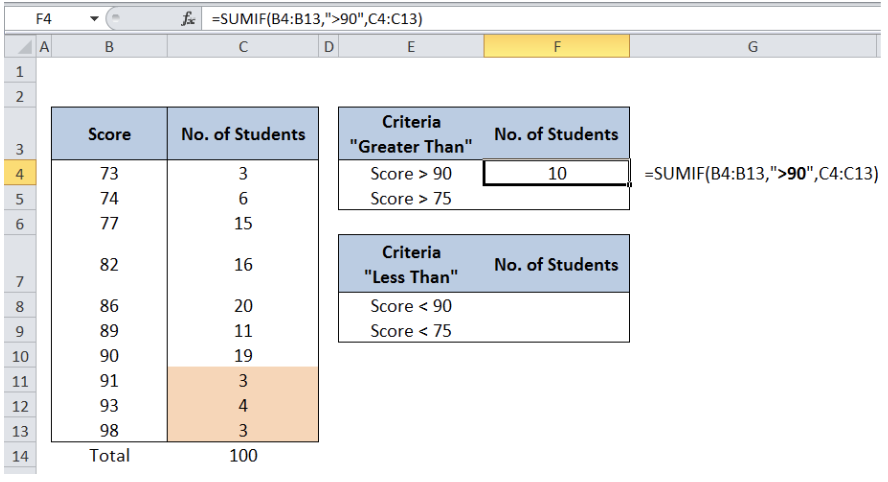Figure 4.  Output: Sum of students with scores greater than 90

### Example 2.

Determine the number of students with scores greater than 75.

Criteria: “>75”

In cell F5, enter the formula =SUMIF(B4:B13,”>75″,C4:C13).

Interpretation: compute the sum if score is greater than 75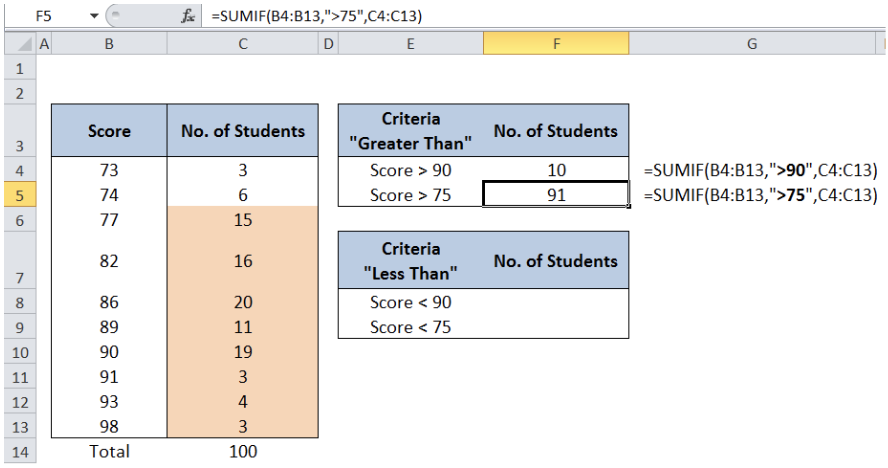Figure 5.  Output: Sum of students with scores greater than 75

The result is 91, which is the sum of the students who scored greater than 75, as highlighted in the cells above.

## Combine SUMIF with Less Than Function

### Example 3:

Determine the number of students with scores less than 90.

Criteria: “<90”

In cell F8, enter the formula =SUMIF(B4:B13,”<90″,C4:C13).

Interpretation: compute the sum if score is less than 90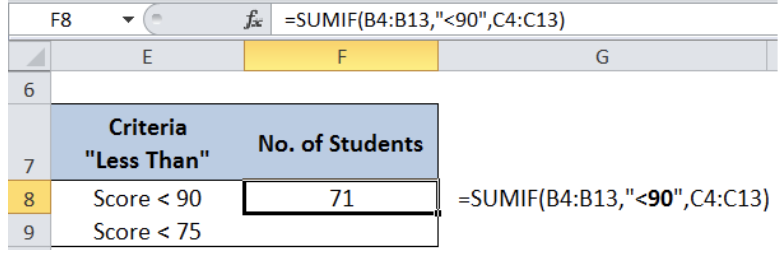Figure 6.  Entering the formula with SUMIF and Less Than Function

The result is 71, which is the sum of the students who scored less than 90, as highlighted in the cells below.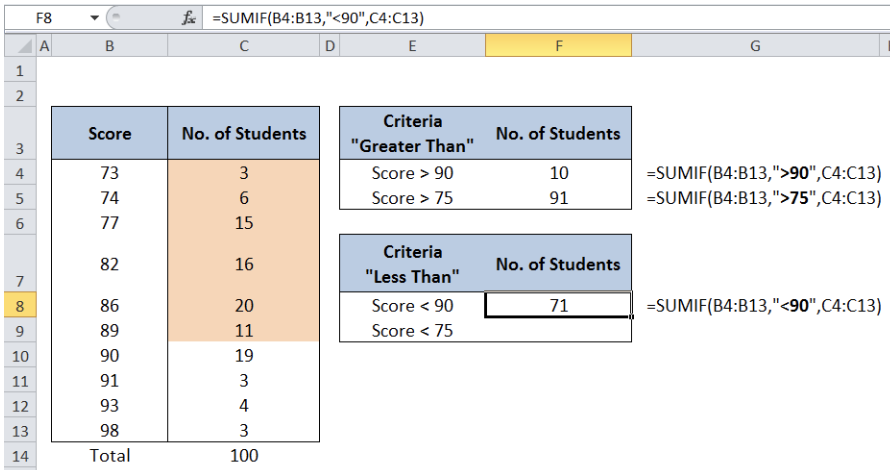Figure 7.  Output: Sum of students with scores less than 90

### Example 4:

Determine the number of students with scores less than 75.

Criteria: “<75”

In cell F9, enter the formula =SUMIF(B4:B13,”<75″,C4:C13).

Interpretation: compute the sumif score is less than 75

The result is 9, as shown below.Figure 8.   Output: Sum of students with scores less than 75

Most of the time, the problem you will need to solve will be more complex than a simple application of a formula or function. If you want to save hours of research and frustration, try our live Excelchat service! Our Excel Experts are available 24/7 to answer any Excel question you may have. We guarantee a connection within 30 seconds and a customized solution within 20 minutes.

### Did this post not answer your question? Get a solution from connecting with the expert.Another blog reader asked this question today on Excelchat:
Solution examplesamending a formula so that there is another criteria and if it does not meet this criteria then we stick with the original criteria
Solved by A. B. in 40 minsI need Column D to = Column C - Column A only if Column B has a 'Date' entered.
Solved by I. B. in 11 minsHello, I need help trying to create a sum of numerical values on a spreadsheet in which the only values that apply to the sum are when the status of another cell is a certain value (e.g. value is not in sum when status: closed, value is in sum when status: open)
Solved by T. W. in 18 minsI am looking for a formula to SUM column L, if Column A matched the relevant date and column C matches the name
Solved by Z. Y. in 16 minsI need a formula that is essentially saying IF this range of cells contain a certain name, add the number in another cell on the same row, and them sum that number in a different cell..!
Solved by O. H. in 44 mins## Subscribe to Excelchat.coAnother blog reader asked this question today on Excelchat: